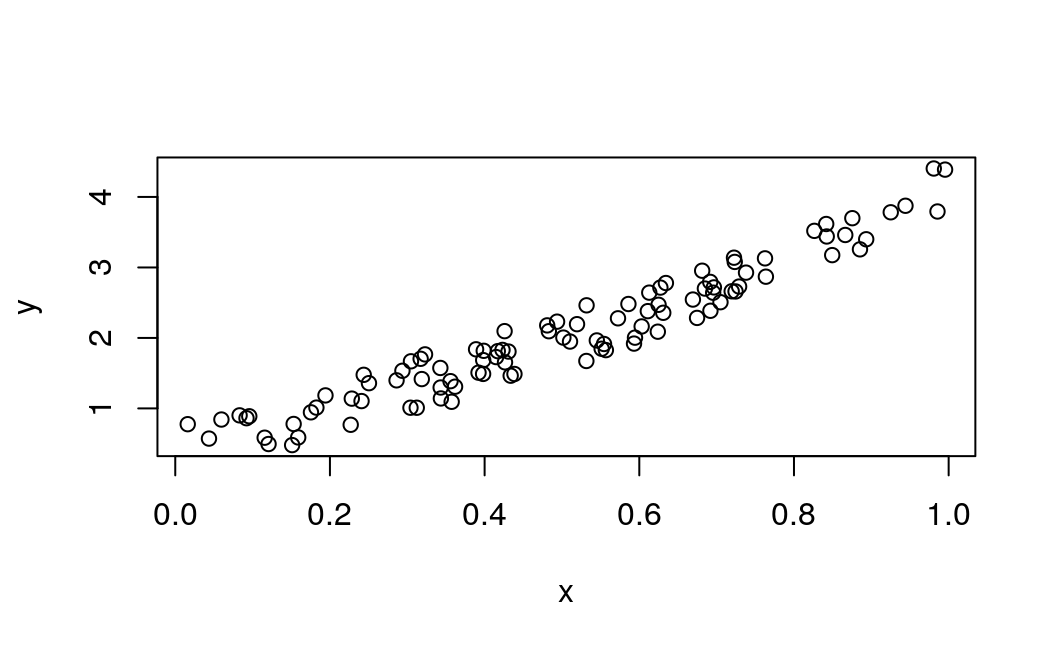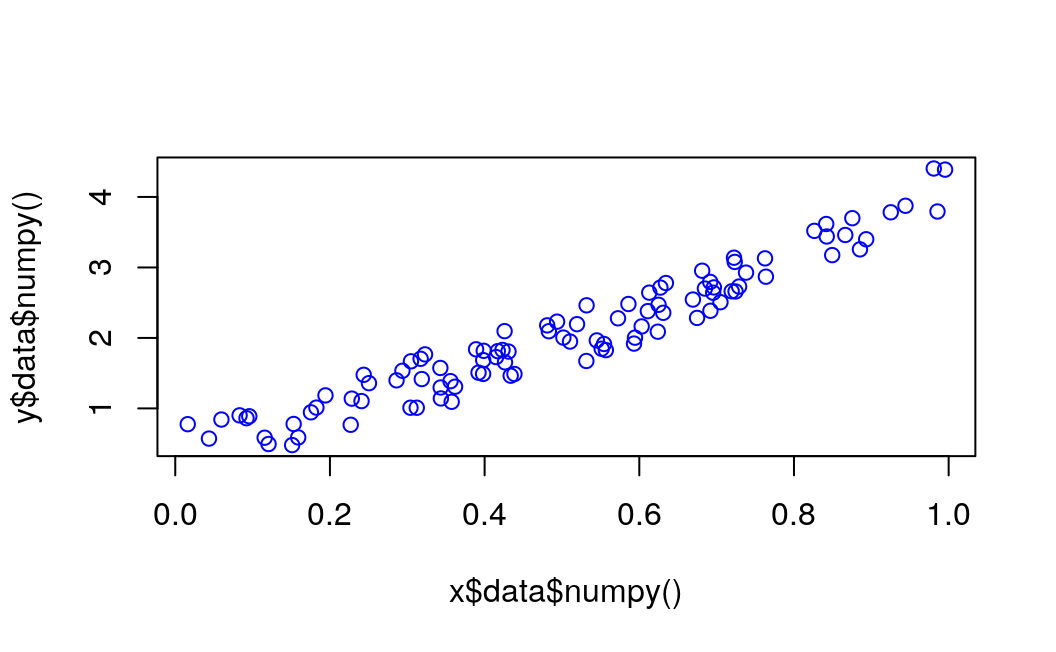# Chapter 10 Linear Regression

Last update: Thu Oct 22 16:46:28 2020 -0500 (54a46ea04)

## 10.1 Introduction

library(rTorch)

nn       <- torch$nn Variable <- torch$autograd$Variable invisible(torch$manual_seed(123))

## 10.2 Generate the dataset

Before you start the training process, you need to know our data. You make a random function to test our model. $$Y = x3 sin(x)+ 3x+0.8 rand(100)$$

np$random$seed(123L)

x = np$random$rand(100L)
y = np$sin(x) * np$power(x, 3L) + 3L * x + np$random$rand(100L) * 0.8

plot(x, y)## 10.3 Convert arrays to tensors

Before you start the training process, you need to convert the numpy array to Variables that supported by Torch and autograd.

## 10.4numpy array to tensor

Notice that before converting to a Torch tensor, we need first to convert the R numeric vector to a numpy array:

# convert numpy array to tensor in shape of input size
x <- r_to_py(x)
y <- r_to_py(y)
x = torch$from_numpy(x$reshape(-1L, 1L))$float() y = torch$from_numpy(y$reshape(-1L, 1L))$float()
print(x, y)
#> tensor([[0.6965],
#>         [0.2861],
#>         [0.2269],
#>         [0.5513],
#>         [0.7195],
#>         [0.4231],
#>         [0.9808],
#>         [0.6848],
#>         [0.4809],
#>         [0.3921],
#>         [0.3432],
#>         [0.7290],
#>         [0.4386],
#>         [0.0597],
#>         [0.3980],
#>         [0.7380],
#>         [0.1825],
#>         [0.1755],
#>         [0.5316],
#>         [0.5318],
#>         [0.6344],
#>         [0.8494],
#>         [0.7245],
#>         [0.6110],
#>         [0.7224],
#>         [0.3230],
#>         [0.3618],
#>         [0.2283],
#>         [0.2937],
#>         [0.6310],
#>         [0.0921],
#>         [0.4337],
#>         [0.4309],
#>         [0.4937],
#>         [0.4258],
#>         [0.3123],
#>         [0.4264],
#>         [0.8934],
#>         [0.9442],
#>         [0.5018],
#>         [0.6240],
#>         [0.1156],
#>         [0.3173],
#>         [0.4148],
#>         [0.8663],
#>         [0.2505],
#>         [0.4830],
#>         [0.9856],
#>         [0.5195],
#>         [0.6129],
#>         [0.1206],
#>         [0.8263],
#>         [0.6031],
#>         [0.5451],
#>         [0.3428],
#>         [0.3041],
#>         [0.4170],
#>         [0.6813],
#>         [0.8755],
#>         [0.5104],
#>         [0.6693],
#>         [0.5859],
#>         [0.6249],
#>         [0.6747],
#>         [0.8423],
#>         [0.0832],
#>         [0.7637],
#>         [0.2437],
#>         [0.1942],
#>         [0.5725],
#>         [0.0957],
#>         [0.8853],
#>         [0.6272],
#>         [0.7234],
#>         [0.0161],
#>         [0.5944],
#>         [0.5568],
#>         [0.1590],
#>         [0.1531],
#>         [0.6955],
#>         [0.3188],
#>         [0.6920],
#>         [0.5544],
#>         [0.3890],
#>         [0.9251],
#>         [0.8417],
#>         [0.3574],
#>         [0.0436],
#>         [0.3048],
#>         [0.3982],
#>         [0.7050],
#>         [0.9954],
#>         [0.3559],
#>         [0.7625],
#>         [0.5932],
#>         [0.6917],
#>         [0.1511],
#>         [0.3989],
#>         [0.2409],
#>         [0.3435]])

## 10.5 Creating the network model

Our network model is a simple Linear layer with an input and an output shape of one.

And the network output should be like this

Net(
(hidden): Linear(in_features=1, out_features=1, bias=True)
)
py_run_string("import torch")
main = py_run_string(
"
import torch.nn as nn

class Net(nn.Module):
def __init__(self):
super(Net, self).__init__()
self.layer = torch.nn.Linear(1, 1)

def forward(self, x):
x = self.layer(x)
return x
")

# build a Linear Rgression model
net <- main$Net() print(net) #> Net( #> (layer): Linear(in_features=1, out_features=1, bias=True) #> ) ## 10.6 Optimizer and Loss Next, you should define the Optimizer and the Loss Function for our training process. # Define Optimizer and Loss Function optimizer <- torch$optim$SGD(net$parameters(), lr=0.2)
loss_func <- torch$nn$MSELoss()
print(optimizer)
print(loss_func)
#> SGD (
#> Parameter Group 0
#>     dampening: 0
#>     lr: 0.2
#>     momentum: 0
#>     nesterov: False
#>     weight_decay: 0
#> )
#> MSELoss()

## 10.7 Training

Now let’s start our training process. With an epoch of 250, you will iterate our data to find the best value for our hyperparameters.

# x = x$type(torch$float)   # make it a a FloatTensor
# y = y$type(torch$float)

# x <- torch$as_tensor(x, dtype = torch$float)
# y <- torch$as_tensor(y, dtype = torch$float)

inputs  = Variable(x)
outputs = Variable(y)

# base plot
plot(x$data$numpy(), y$data$numpy(), col = "blue")
for (i in 1:250) {
prediction = net(inputs)
loss = loss_func(prediction, outputs)
optimizer$zero_grad() loss$backward()
optimizer$step() if (i > 1) break if (i %% 10 == 0) { # plot and show learning process # points(x$data$numpy(), y$data$numpy()) points(x$data$numpy(), prediction$data$numpy(), col="red") # cat(i, loss$data\$numpy(), "\n")
}
}## 10.8 Results

As you can see, you successfully performed regression with a neural network. Actually, on every iteration, the red line in the plot will update and change its position to fit the data. But in this picture, you only show you the final result.